Page top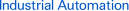# FAQ01222 of Displacement Sensors / Measurement Sensors FAQ

FAQ No. FAQ01222

Question

What is average processing (moving average)?

﻿

The average processing performed by the Smart Sensor uses a moving average. A moving average is an average where each time a sample is taken the average of the last n samples including the current sample is calculated and displayed. Therefore, the average is associated with the preceding data.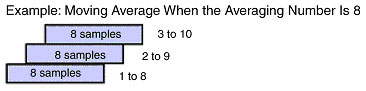The average processing performed by the Smart Sensor uses a moving average. A moving average is an average where each time a sample is taken the average of the last n samples including the current sample is calculated and displayed. Therefore, the average is associated with the preceding data.

Select the averaging number as required by the application.

The averaging number of the Smart Sensor can be set to 1, 2, 4, 8, 16, 32, 64, 128, 256, 512, 1024, 2048, or 4096 samples.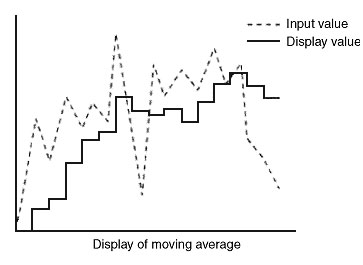Reference Explanation:

A simple average is shown below. (Remember, the Smart Sensor uses a moving average as described above, not a simple average.) You will be able to see the meaning (effectiveness) of the moving average by comparing it to a simple average.

A simple average takes n samples and calculates the average of those values. The display of the average remains the same until the next nth sampling is taken. It is simply the average of n samples so it has nothing to do with the previous data.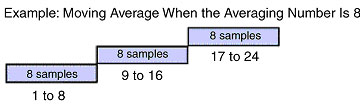The output response is slower for simple average.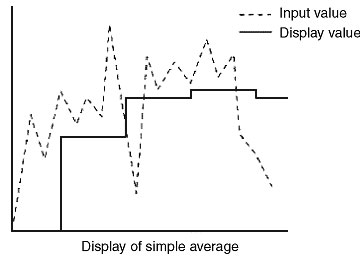## Recommended Products

###E2EStandard Sensors for Detecting Ferrous Metals under Standard Conditions